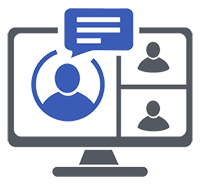## Introduction to R & Statistical Modelling in R

Date:

13/11/2023 - 16/11/2023

Organised by:

Royal Statistical Society

Presenter:

Jumping Rivers

Level:

Entry (no or almost no prior knowledge)

Contact:Venue: Online

### Description:

Level: Foundation (F)

The purpose of this course is to introduce participants to the R environment for statistical computing. The course focuses on entering, working with and visualising data in R, and linear regression modelling in R.

Learning Outcomes

By the end of the course, participants will be able to use R to:

• Direct themselves around the R interface in an efficient way
• Import and export their own data from spreadsheets and a number of other data storages to R
• Summarise the data with R's built-in summary statistic functions
• Plot data in interesting ways
• manipulate data in ways such that they can efficiently analyse data
• Have a thorough understanding of popular statistical techniques
• Have the skills to make appropriate assumptions about the structure of the data and check the validity of these assumptions in R
• Be able to fit regression models in R between a response variable
• Understand how to apply said techniques to their own data using R's common interface to statistical functions
• Be able to cluster data using standard clustering techniques

Topics Covered

• Introduction to R: A brief overview of the background and features of the R statistical programming system
• Data entry: A description of how to import and export data from R
• Data types: A summary of R's data types
• R environment: A description of the R environment including the R working directory, creating/using scripts, saving data and results
• R graphics: Creating, editing and storing graphics in R
• Summary statistics: Measures of location and spread
• Manipulating data in R: Describing how data can be manipulated in R using logical operators
• Vector operations: Details of R's vectors operations
• Basic hypothesis testing: Examples include the one-sample t-test, one-sample Wilcoxon signed-rank test, independent two-sample t-test, Mann-Whitney test,teo-sample t-test for paired samples. Wilcoxon signed-rank test
• ANOVA tables: One-way and two-way tables
• Simple and multiple linear regression: Including model diagnostics
• Clustering: Hierarchical clustering, k-means
• Principle components analysis: Plotting and scaling data

Target Audience

This course is ideally suited to anyone who:

• Is familiar with basic statistical methods (e.g. t-tests, boxplots) and who want to implement these methods using R
• Has used menu-driven statistical software (e.g. SPSS, Minitab) and who want to investigate the flexibility offered by a command line package such as R
• Is already familiar with basic statistical methods in R and would like to extend their knowledge to regression involving multiple predictor variables, binary, categorical and survival response variables
• Is familiar with regression methods in menu-driven software (e.g. SPSS, Minitab) and who wish to migrate to using R for their analyses

Assumed Knowledge

The course requires familiarity with basic statistical methods (e.g. t-tests, box plots) but assumes no previous knowledge of statistical computing.

For this online course, participants are not required to have R installed on their own laptops. A virtual environment, which can be accessed through a web browser, will be used to run R and view course materials.

Cost:

From £629.75 to £873.94 (including VAT)

Website and registration:

Region:

Greater London

Keywords:

Quantitative Data Handling and Data Analysis, R, Visualising Data, Linear regression,

Related publications and presentations:

Back to archive...